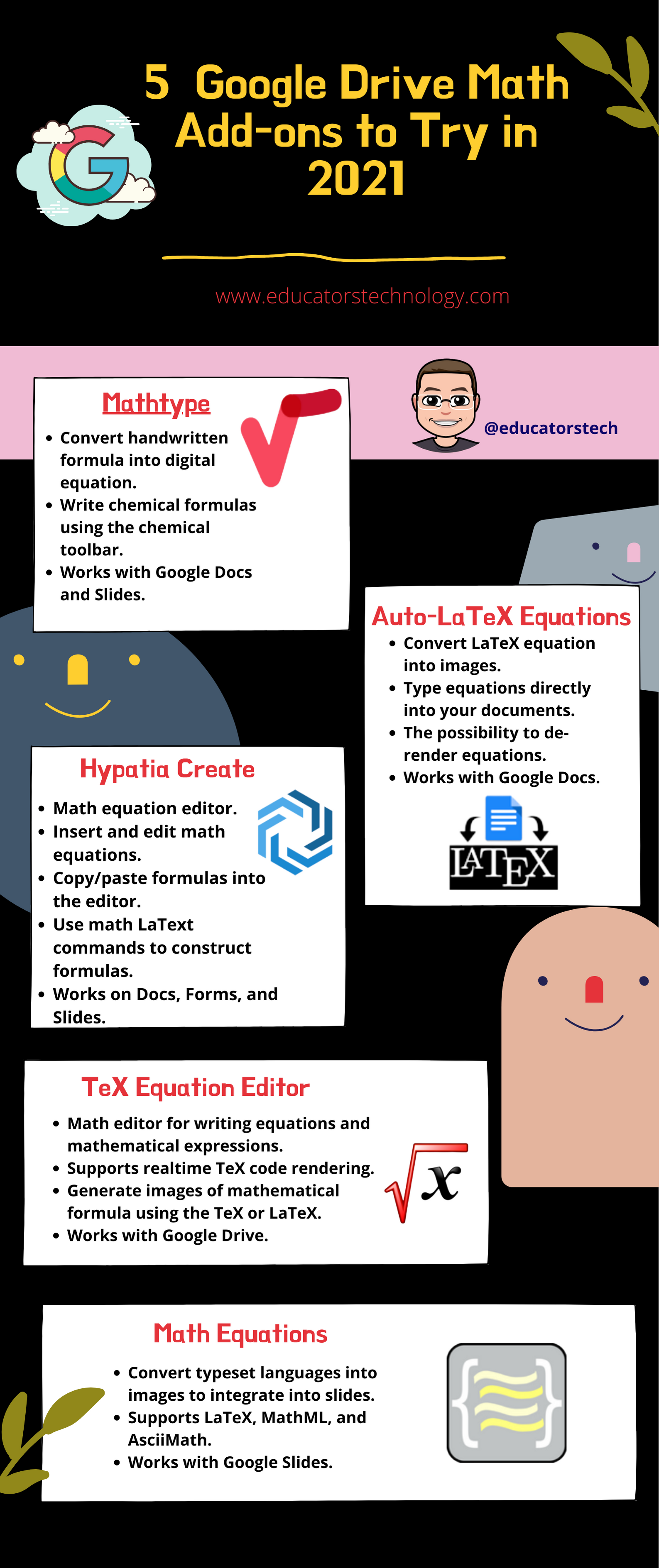Below is a collection of some of the best math add-ons that work with various Google Drive services. Most of them are math editors that you and your students can use to carry out complex mathematical equations and write different mathematical expressions and characters into your docs, slides, and/or sheets. I invite you to check them out and share with us your feedback on our social media profiles.

MathType
• Convert handwritten formula into digital equation.
• Write chemical formulas using the chemical toolbar.
• Works with Google Docs and Slides.
Hypatia Create
• Math equation editor.
• Insert and edit math equations.
• Copy/paste formulas into the editor.
• Use math LaText commands to construct formulas.
• Works on Docs, Forms, and Slides.
Auto-LaTeX Equations
1. Convert LaTeX equation into images.
2. Type equations directly into your documents.
3. The possibility to de-render equations.